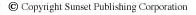After mapping out your home’s wiring circuits (see How to Map House Electrical Circuits), the next step is to determine your present usage, or electrical load. This would be a time-consuming task if you had to go around the house and add up all the wattages of the lights and appliances; however, the National Electrical Code (NEC) has established certain values that represent typical electrical usage.

Three watts per square foot of existing living space and space for future use is used to figure electrical load for general-purpose circuits (lighting and receptacles). A nominal value of 1,500 watts is used for each 20-amp small-appliance circuit (circuits that power receptacles in the kitchen, dining room, family room, breakfast room, and pantry) and for a laundry circuit.

By applying these values to your home and using the actual nameplate values affixed to major appliances, you can use a handy formula to calculate your electrical load.

Consider the example of a house with 1,800 square feet (based on outside dimensions) of finished living space and space adaptable for future use. The house has the usual two small-appliance circuits (3,000 watts), a laundry circuit (1,500 watts), a hot water heater (5,500 watts), a clothes dryer (5,600 watts), a dishwasher (1,500 watts), a garbage disposal (600 watts), a range (15,000 watts), and a central air conditioner (5,000 watts).

The first step is to multiply 1,800 square feet by 3 watts per square foot. The total is 5,400 watts for lighting and general-purpose circuits. Add 3,000 watts for the two small-appliance circuits and 1,500 watts for the laundry circuit for a total of 9,900 watts. Next, add the values of all the major appliances, except the central air conditioner, for a total of 38,100 watts.

The next step is to figure 40 percent of the amount over 10,000 watts (0.40 x 28,100 = 11,240 watts). Adding the 10,000 watts to the 11,240 watts gives a subtotal of 21,240 watts. Then add the 5,000 watts of the central air conditioner for a grand total of 26,240 watts. This is your estimated load in watts.

To figure the current needed to carry that load, divide 26,240 watts by 240 volts. The total comes to 109.33 amps; therefore, of the standard service ratings (60, 100, 125, 150, and 200), the rating for this sample house should be 125 amps or higher.

Now try it yourself for your own home. Then compare the total load in amps with your present service rating. If the two values are close together, your present service cannot handle the addition of many new loads.

If your service rating is less than 100 amps, you can’t use this formula to calculate your load. You can, however, use a different formula that incorporates the same NEC values for typical electrical usage. The general-purpose circuits, small-appliance circuits, and laundry circuits are computed exactly as they are in the first formula.

Once you’ve figured the general-purpose circuit load (3 watts x number of square feet of living area), add 1,500 watts for each 20-amp small-appliance circuit and laundry circuit. Using this total, add 100 percent of the first 3,000 watts and 35 percent of the balance over 3,000 watts [3,000 + 0.35 (total – 3,000)].

Add to this value the nameplate ratings of all major appliances (space heater, garbage disposal, dishwasher, etc.). This gives you your estimated load in watts. You can find the current by dividing the total wattage by your voltage—120 volts for two-wire service and 240 volts for three-wire service.